Next: Three Spring-Coupled Masses Up: Coupled Oscillations Previous: Two Spring-Coupled Masses

# Two Coupled LC Circuits

Consider the LC circuit pictured in Figure 17. Let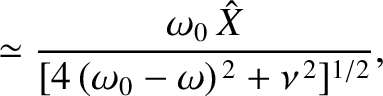,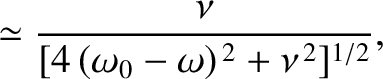, and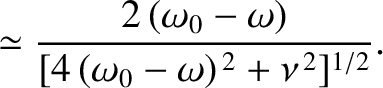be the currents flowing in the three legs of the circuit, which meet at junctionsand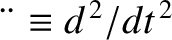. According to Kirchhoff's first circuital law, the net current flowing into each junction is zero (Grant and Phillips 1975). It follows that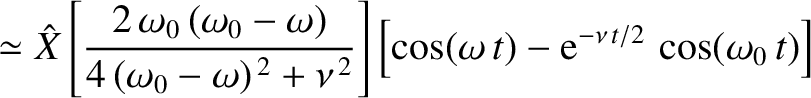. We deduce that this is a two degree of freedom system whose instantaneous configuration is specified by the two independent variablesand. It follows that there are two independent normal modes of oscillation. The potential drops across the left, middle, and right legs of the circuit are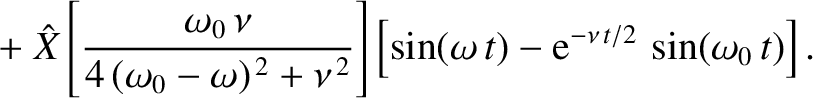,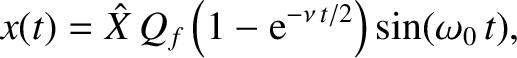, and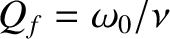, respectively, where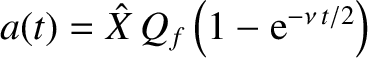,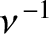, and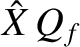. However, because the three legs are connected in parallel, the potential drops must all be equal, so that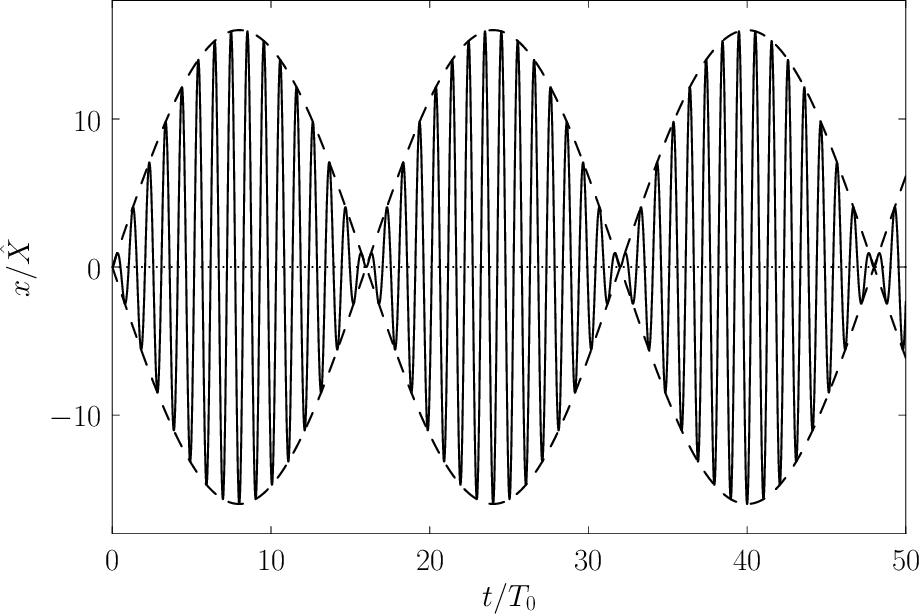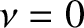(182)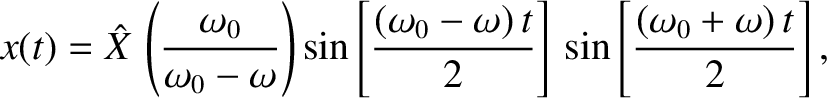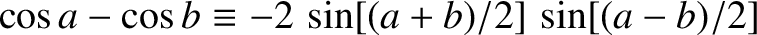(183)

Differentiating with respect to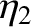, and dividing by, we obtain the coupled time evolution equations of the system: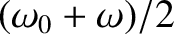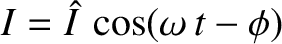(184)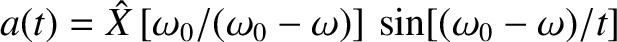(185)

where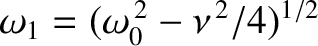and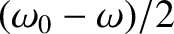.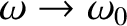It is fairly easy to guess that the normal coordinates of the system are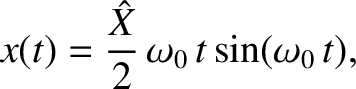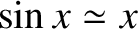(186)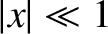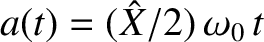(187)

Forming the sum and difference of Equations (184) and (185), we obtain the evolution equations for the two independent normal modes of oscillation,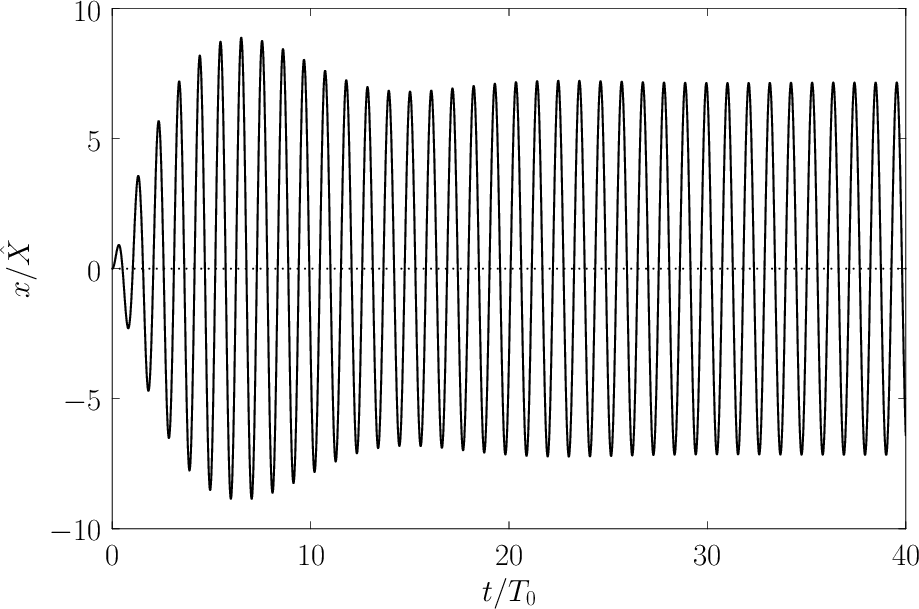(188)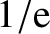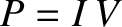(189)

(We can be sure that we have correctly guessed the normal coordinates because the previous two equations do not couple to one another.) These equations can readily be solved to give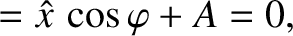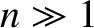(190)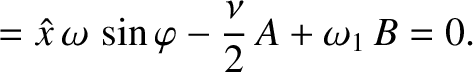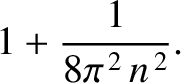(191)

where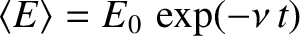. Here,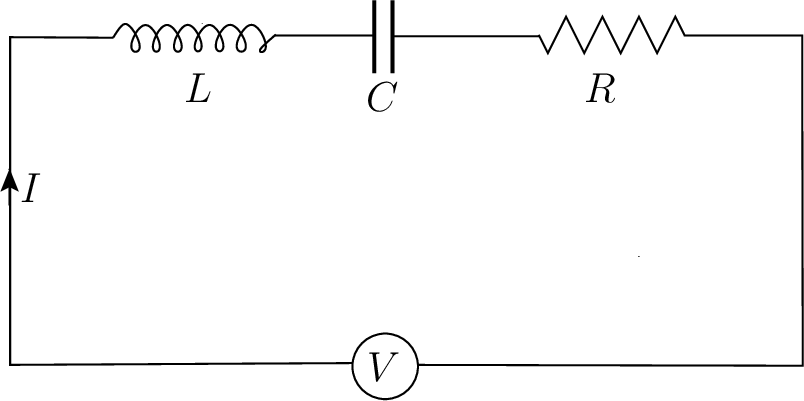,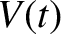,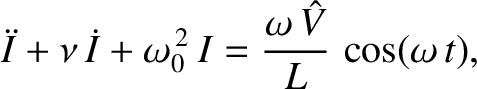, and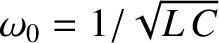are constants determined by the initial conditions. It follows that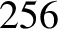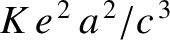(192)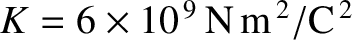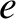(193)

As an example, suppose that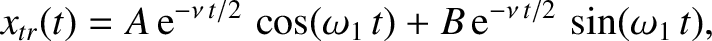and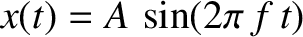. We obtain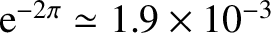(194)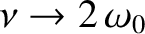(195)

where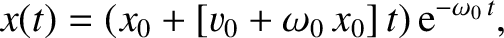. This solution is illustrated in Figure 18. [Here,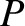and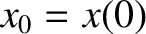. Thus, the two normal frequencies are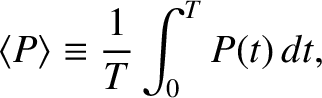and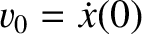.] Observe the beat modulation generated by the superposition of two normal modes with similar normal frequencies. (See Section 3.6.)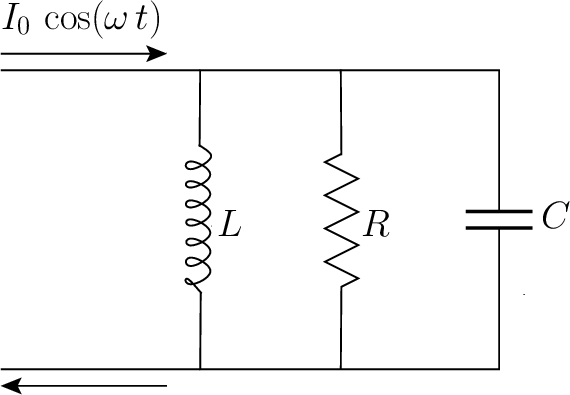We can also solve the problem in a more systematic manner by specifically searching for a normal mode of the form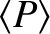(196)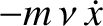(197)

Substitution into the time evolution equations (184) and (185) yields the matrix equation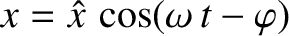(198)

where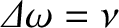. The normal frequencies are determined by setting the determinant of the matrix to zero. This gives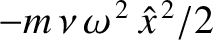(199)

or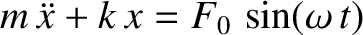(200)

The roots of the preceding equation are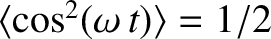and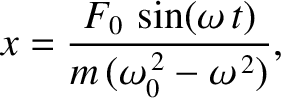. (Again, we have neglected the negative frequency roots, because they generate the same patterns of motion as the corresponding positive frequency roots.) Hence, the two normal frequencies areand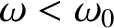. The characteristic patterns of motion associated with the normal modes can be calculated from the first row of the matrix equation (198), which can be rearranged to give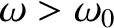(201)

It follows that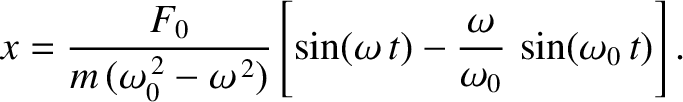for the normal mode with, and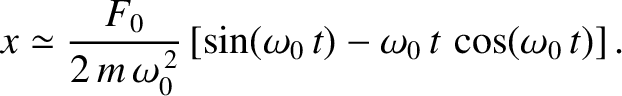for the normal mode with. We are thus led to Equations (192) and (193), whereandare the amplitude and phase of the higher frequency normal mode, whereasandare the amplitude and phase of the lower frequency mode.Next: Three Spring-Coupled Masses Up: Coupled Oscillations Previous: Two Spring-Coupled Masses
Richard Fitzpatrick 2013-04-08